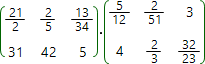Matrices multiplication online calculator

Multiplication matrix A by matrix B can be applied if and only if the number of columns of matrix A equal to the number of rows of matrix B. The result matrix dimensions: number of rows equal to the number of rows of matrix A and number of columns equal to the number of columns of matrix B:

An × mBm × k = Cn × k

Matrices multiplication is noncommutative operation:

ABBA

Matrix multiplication calculator
A: ×
B: ×A =
B =